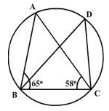In the figure, angle ABC = 65° and angle ACB = 58°, the measure of angle BDC is• a)
45°
• b)
68°
• c)
90°
• d)
57°

### Related TestJohan Joseph Mar 01, 2022
angle ABC = 65�

angle ACB = 58�

In traingle  BAC
angle A = x
angle A  = 65
+58
+x = 180
( angle sum property)
x = 180 - 123
x = 57
so ,  angle A  = 57
{ Angle BDC = 57
� ( angles in a same segment are equal ) theorem }

angle ABC = 65�angle ACB = 58�In traingle BACangle A = xangle A = 65�+58�+x = 180�( angle sum property)x = 180 - 123x = 57�so , angle A = 57�{ Angle BDC = 57� ( angles in a same segment are equal ) theorem }